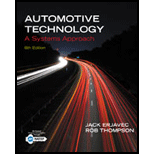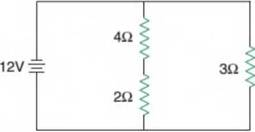Chapter 15, Problem 3ASRQAutomotive Technology: A Systems A...

6th Edition
Jack Erjavec + 1 other
ISBN: 9781133612315

Solutions

Chapter
SectionAutomotive Technology: A Systems A...

6th Edition
Jack Erjavec + 1 other
ISBN: 9781133612315
Textbook Problem

WHiile discussing the circuit shown in Figure 15-63: Technician A says that the total resistance of the circuit is 1 ohm. Technician B says that the current flow through the 6-ohm resistor is 12 amps. Who is correct?Figure 15-63 Technician A Technician BBoth A and BNeither A nor B

To determine

The correct argument between the technicians

Explanation

Given:

Technician A says that the total resistance of the given circuit is 1 ohm.

Technicians B say that the current flow through the 6 ohm resistor is 12 amps.

Concept used:

Series circuits: When resistors are in series, then equivalent resistance is the algebraic sum of the individual resistances.

Req=R1+R2

Parallel circuits: When resistors are connected in parallel, then the reciprocal of equivalent resistance is equal to the algebraic sum of the reciprocals of individual resistances.

1Req=1R1+1R2

In parallel connections, the voltage remains the same.

And also, current i = vr

Where, v= voltage

r= resistance

Still sussing out bartleby?

Check out a sample textbook solution.

See a sample solution

The Solution to Your Study Problems

Bartleby provides explanations to thousands of textbook problems written by our experts, many with advanced degrees!

Get Started

How many classes of fit are there in the Unified National system?

Precision Machining Technology (MindTap Course List)

Express the angular speed of the earth in rad/s and rpm.

Engineering Fundamentals: An Introduction to Engineering (MindTap Course List)

Convert 10,000 ft-lbf of energy into BTU, Joules, and kilojoules.

Fundamentals of Chemical Engineering Thermodynamics (MindTap Course List)

Same as Problem 8.4-19, but use ASD.

Steel Design (Activate Learning with these NEW titles from Engineering!)